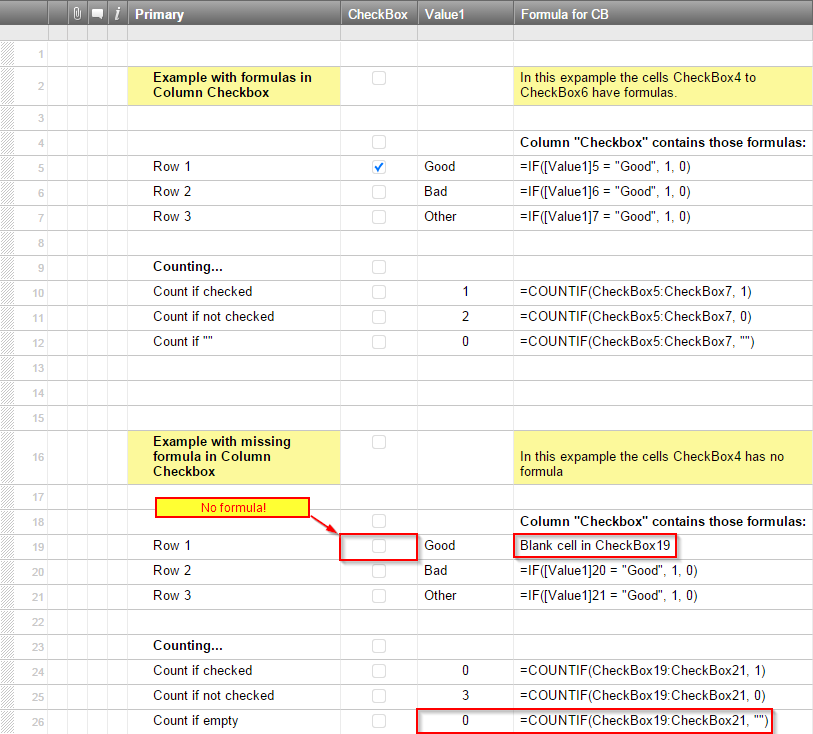#### Welcome to the Smartsheet Forum Archives

The posts in this forum are no longer monitored for accuracy and their content may no longer be current. If there's a discussion here that interests you and you'd like to find (or create) a more current version, please Visit the Current Forums.

# Calculate with row numbers / Formulas not copied after adding new rows

Every now and then I notice that formulas are not copied when a new row is added to a sheet. Very often these formulas are placed in hidden columns and so it's not immediately obvious.

So, I'm looking for a way to get an "alert" in a cell within Smartsheet whenever a cell in a specific range is empty, showing the amount of blank cells. I know the first and the last row of the range, but the count of rows in between can vary...

I could manage to get the count of cells with a specific content within a given range, but if I want to be alerted, I need a value to compare the result with...

Total amount of rows=5

Total amount of non blank cells=4

In Excel one can calculate with a row number with the following function:

=row()

This formula returns the number of the row. One can use it to count the number of rows between a specific range. But it seems that Smartsheet does not support this function.

I could not manage to use "count(children())" because children may again have children and with this formula you do not get the grand total of children and grandchildren.

At the moment I'm lost ;-)

Does anyone have a solution or hint how to get this working?

Best,

Dietrich

Tags:

• For counting blank cells, you can use this:

=COUNTIF(Cell1:Cell50, "")

You can reference an entire column by changing it to this:

=COUNTIF(Cell:Cell, "")

• John,

thanks for your tip and it works great - only with Checkbox columns I'm still struggeling...

I use a formula that automatically activates the checkbox (switch to value 1) depending on a value in another cell (same row).  If I take a formula like

=COUNTIF(Cell1:Cell50, "")

it seems to me, that it does not differ between the value 0 and an empty cell.

Example in the picture below...
Cell "Checkbox19 has no formular, but count in Cell "[Value1]26" is zero...

Any idea?

Best

Dietrich• Hi Sorry for taking so long to get back to you!

If you have a formula in the checkbox cell that makes the box unchecked, this will count them:

=COUNTIF(Cell1:Cell50, "")

If you have blank checkboxes without a formula or anything else in it, this will count the unchecked boxes:

=COUNTIF(Cell1:Cell50, 0)

If you have a combination of the two, you can add those two COUNTIF functions together.

• Hmmm,

sorry, looks like I got my wires still crossed ;-)

It seems that the problem is, that even if the cell has no formula, it would be counted as 0, because this is the default value for an empty cell...

Example:

- I have 5 Cells in a column formatted as checkbox.

- All cells have a formula to decide if the value is 1 or 0

- One cell shall have a 1 according to his formula

Now I delete the formula in one of the cells where the regular result of the formula is 0 in the checkbox column > the value of this cell is 0 = empty checkbox.

The formula =count(cell1:cell5)

has 5 as result.

The formula =countif(cell1:cell5, 0)

has 4 as result, since 4 of 5 have the value 0

The formula =countif(cell1:cell5,"")

has 0 as result, since all cells have a value

4 have a formula, one is 0 (=empty by default)

I still can not count the one without formula...

Best

Dietrich

• Right, the =COUNTIF(Cell1:Cell50, "") doesnt count blank cells in checkbox columns, sorry!